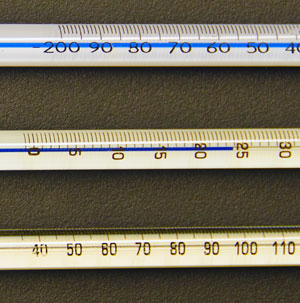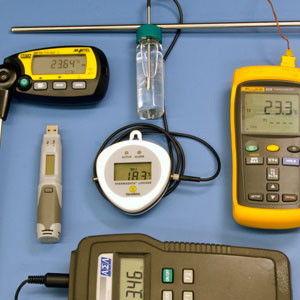# Temperature

#### By Mark Ciotola

First published on May 16, 2019. Last updated on April 29, 2021.

## Introduction

Recall that one form of energy is thermal energy, which comprises the random motion of individual molecules that are part of a larger system. For example, water molecules in a tea kettle are moving back and forth in all directions and at many different speeds. Some water molecules might be moving slowly, and they would have a lower energy than those that are moving quickly.

Temperature refers to the intensity of thermal energy. Regardless of the energy of individual molecules, if their average (mean) velocity is high, then the temperature of the collection of those molecules is high. The the average is low, then the temperature is low.

## Measuring TemperatureAnalog thermometers (source: U.S. govt.)

One cannot easily measure the individual velocities of molecules within a large collection of such. Fortunately, there exists easier ways to measure temperature. The traditional device for measuring temperature is the thermometer. Thermometers can operate by measuring the expansion of a fluid such as mercury or alcohol. Other thermometers operate by comparing the expansion on one metal to another.

More modern devices can measure temperature by detecting infrared radiation emitted from an object. Such devices only measure surface temperature, but adjustments can be made to infer the internal temperature of an object such as a human.

## Units of TemperatureTemperature measuring devices (source: U.S. government)

• In the USA, the degree Fahrenheit, F, is used to express temperature.
• In the metric system, the degree Celsius (or Centigrade)  expresses temperature.
• The preferred unit by physicists is the Kelvin, K. One unit of Kelvin is equal to one degree Celsius, except that the Kelvin system starts at absolute zero temperature. 0 degrees Celsius is equal to 273.15 Kelvin.

## Distinction Between Thermal Energy and Temperature

Thermal energyis the kinetic energy contained in the random movement of molecules. Temperature is the measure of the strengthor intensitysuch thermal energy. Temperature does not concern the amount of thermal energy. For example, a cup of water may be quite hot, yet contain much less thermal energy than the near-freezing Arctic Ocean. The relationship between temperature Tand thermal energy Qis as follows:

$$T = \frac{Q}{MC}$$,

where Mis the mass under consideration and Cis the heat capacity of the substance containing the thermal energy.

## Resource

U.S. National Institute of Standards and Technology (NIST), SI Units: Temperature.